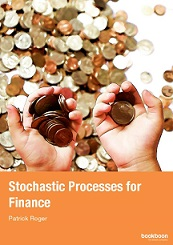Processing ......FreeComputerBooks.com Links to Free Computer, Mathematics, Technical Books all over the World

Stochastic Processes for Finance
Top Free C Programming Books 🌠 - 100% Free or Open Source!
• Title Stochastic Processes for Finance
• Authors Jonathan Block
• Publisher: Bookboon, University of Pennsylvania
• eBook PDF
• Language: English
• ISBN-10: N/A
• ISBN-13: 978-87-7681-666-7Book Description

This book is an extension of Probability for Finance to multi-period financial models, either in the discrete or continuous-time framework. It describes the most important stochastic processes used in finance in a pedagogical way, especially Markov chains, Brownian motion and martingales. It also shows how mathematical tools like filtrations, Ito's lemma or Girsanov theorem should be understood in the framework of financial models. It also provides many illustrations coming from the financial literature.

A wonderful display of the use of mathematical probability to derive a large set of results from a small set of assumptions. In summary, this is a well-written text that treats the key classical models of finance through an applied probability approach. It should serve as an excellent introduction for anyone studying the mathematics of the classical theory of finance.

In recent years, modeling financial uncertainty using Stochastic Processes has become increasingly important, but it is commonly perceived as requiring a deep mathematical background. This book shows that this is not necessarily so. It presents the theory of discrete stochastic processes and their applications in finance in an accessible treatment that strikes a balance between the abstract and the practical. Using an approach that views sophisticated stochastic calculus as based on a simple class of discrete processes - "random walks" - the author first provides an elementary introduction to the relevant areas of real analysis and probability. He then uses random walks to explain the change of measure formula, the reflection principle, and the Kolmogorov backward equation. The Black-Scholes formula is derived as a limit of binomial model, and applications to the pricing of derivative securities are presented.

Another primary focus of the book is the pricing of corporate bonds and credit derivatives, which the author explains in terms of discrete default models.By presenting important results in discrete processes and showing how to transfer those results to their continuous counterparts, this book imparts an intuitive and practical understanding of the subject. This unique treatment is ideal both as a text for a graduate-level class and as a reference for researchers and practitioners in financial engineering, operations research, and mathematical and statistical finance.

Reviews, Ratings, and Recommendations: Related Books and Categories: Read and Download Links:Similar Books:
 :All CategoriesTop Free BooksRecent BooksMiscellaneous BooksComputer EngineeringComputer LanguagesComputer ScienceData Science/DatabasesJava and Java EE (J2EE)Linux and UnixMathematicsMicrosoft and .NETMobile ComputingNetworking and CommunicationsSoftware EngineeringSpecial TopicsWeb Programming